## Gaussian Double Mean Process

We can view double mean as a general process to find means of two input numbers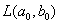using as a recurrence formula of two means.

Philips (2000) proposed Gaussian double mean process is a difference equation that involves two means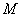and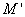with two inputs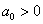and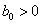, which relates to each other using the following formula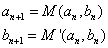Where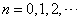Compare this difference equation formula with Archimedean Double mean process .

The Gaussian double mean has a very nice property that the sequence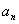and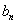will converge to a common limitand it converges very fast with quadratic speed.

Example: (Arithmetic Geometric mean)

We use arithmetic and geometric mean, thus the name of this mean is AGM = Arithmetic Geometric mean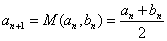and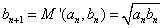Philips (2000) shows that the sequenceandwill converge to a common limitwhere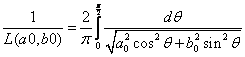For instance: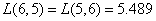,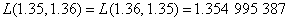You may notice that AGM is symmetric mean.

Example: ( Harmonic-geometric mean )

We use harmonic mean and geometric mean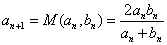and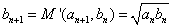Compare this with the Archimedean harmonic-geometric mean, the (Gaussian) Harmonic-geometric mean is symmetric.

For instance: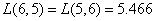,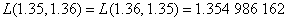Preferable reference for this tutorial is

Teknomo, Kardi (2015) Mean and Average. https:\\people.revoledu.com\kardi\tutorial\BasicMath\Average\